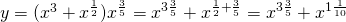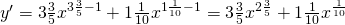# Solution assignment 21 Differentiation of standard functions

### Assignment 21

Differentiate:### Solution

This seems a complicated function and indeed, it is more difficult than the previous functions. However, recall that a root function can be written as a power function. In this case the function can be rewritten as:This is the sum of two power functions and thus the derivative is:0